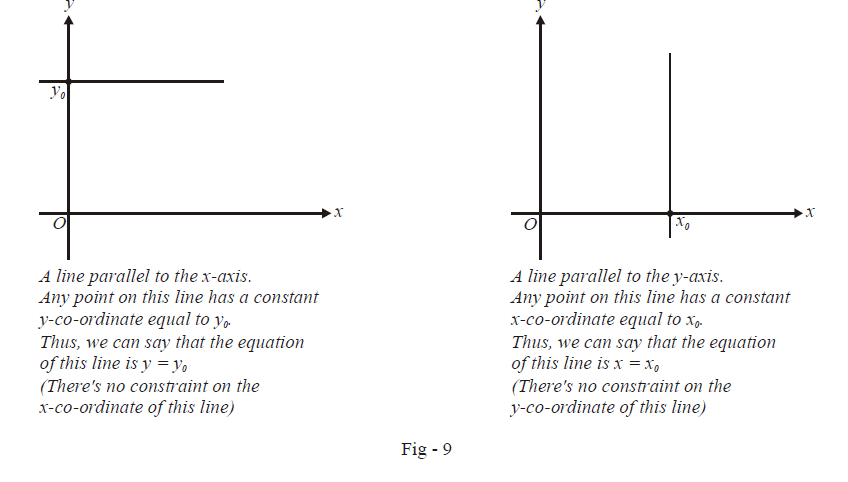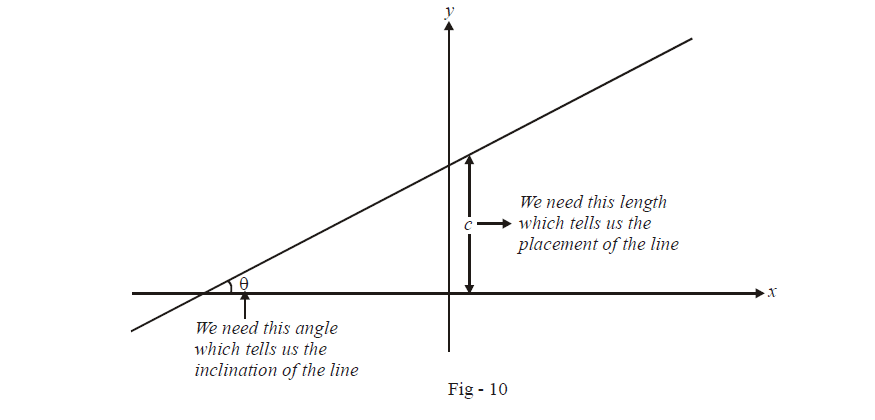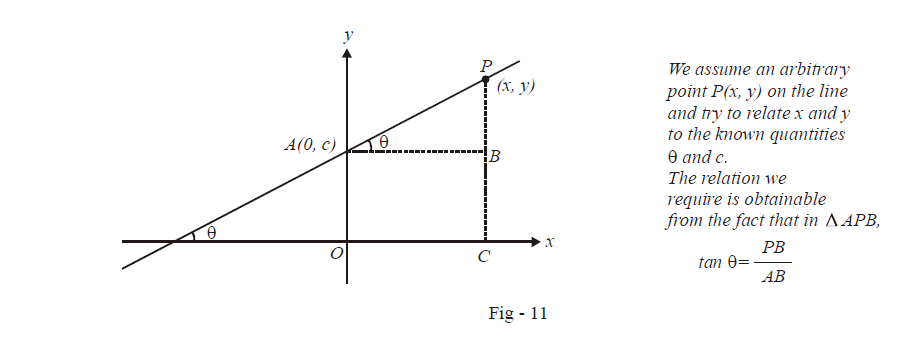# Slope Intercept Form of a Straight Line Equation

Go back to  'Straight Lines'

$$\textbf{Art 4 :} \qquad\boxed{{\text{Equation(s) representing a straight line}}}$$

The last three articles dealt with the preliminaries of co-ordinate geometry and certain elementary formulae which find widespread use. With this article, we start the discussion of the geometry of straight lines in detail.

On the co-ordinate plane, the simplest case for a straight line would be one in which the line is parallel to one of the co-ordinate axes.As described in the figure above, the equation of such a line is $$y={{y}_{0}}\,\,\text{or}\,\,x={{x}_{0}}$$ accordingly as the line is parallel to the x-axis or the y-axis respectively.

These are special cases of lines; we want to find the equation of any arbitrary line in general. Visualise any such line in your mind. To completely specify such a line, you would need two quantities: the inclination of the line (or its slope or the angle it makes with say, the x-axis) and the placement of the line (i.e. where the line passes through with reference to the axes: we can specify the placement of the line by specifying the point on the y-axis through which the line passes, or in other words, by specifying the y-intercept.)It should be obvious to you that any line can be determined uniquely using these two parameters.

We now find out the equation of this straight line, assuming that we know $$\theta \,\,\text{and}\,\,c.$$  In other words, we intend to find out the relation that the co-ordinates (x, y) of any arbitrary point on the line must satisfy. The determination of this equation is straightforward:As described in the figure above, we have in $$\Delta \,APB,$$

$\tan \theta =\frac{PB}{AB}$

$$\tan \theta$$ is a measure of the inclination of the line (its steepness). $$\tan \theta$$  is therefore termed the slope of the line and is denoted by m. Thus, $$m = \tan \theta$$ . Also, notice that $$PB=\left( y-c \right)\,\,\text{and}\,\,AB=x.$$ Therefore,

\begin{align} & \qquad\qquad m = \frac{{y - c}}{x}\\& \Rightarrow \qquad \boxed{y\,\,\,\, = \,\,\,\,mx\,\,\, + \,\,\,\,c}\,\,\,\,\,\,\,\,\,\,\,\,\,\,\,\,\,\,\,\,\,:{\;\mathbf{Slope}}{\text{ }} - {\text{ }}{\mathbf{intercept}}{\text{ }}{\mathbf{form}} \\&\qquad\qquad\quad\quad\nearrow \qquad\nearrow \,\,\,\,\, \\&\qquad\qquad{\text{Slope}}\,\,\,\,\,\,\,\,\,\,\,y - {\text{intercept}}\,\,\,\end{align}

This is the general equation of a straight line involving its slope and its y-intercept. This form of the equation of the line is therefore termed the slope-intercept form.

Notice that if the line passes through the origin, its equation would reduce to y = mx.

As you might have guessed by now, this is not the only form to represent a straight line. This form uses the slope and the intercept of the line.